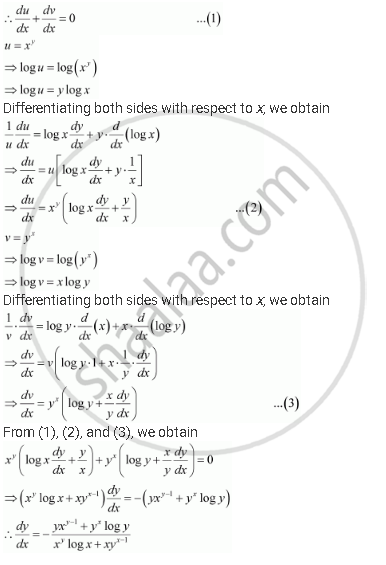# Find Dy/Dx of Function Xy + Yx = 1 - Mathematics

#### Question

Find dy/dx of function

xy + yx = 1

#### Solution

The given function is xy + yx = 1

Let xy = u and yx = v

Then, the function becomes u v = 1Is there an error in this question or solution?

#### APPEARS IN

NCERT Solution for Mathematics Textbook for Class 12 (2018 (Latest))
Chapter 5: Continuity and Differentiability
Q: 12 | Page no. 178

#### Video TutorialsVIEW ALL 

Find Dy/Dx of Function Xy + Yx = 1 Concept: Logarithmic Differentiation.